Question #4797
consider the function F= x^2 | x+2| for x&lt;-2 (2/x)+1 for -2 &lt;or= x &lt; 0 3 for x=o (sinx/x) for x&gt;0 describe all asymptotes of F (vertical and horizontal)
Asymptote of a curve is a line such that the distance between the curve and the line approaches zero as they tend to infinity.We have function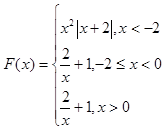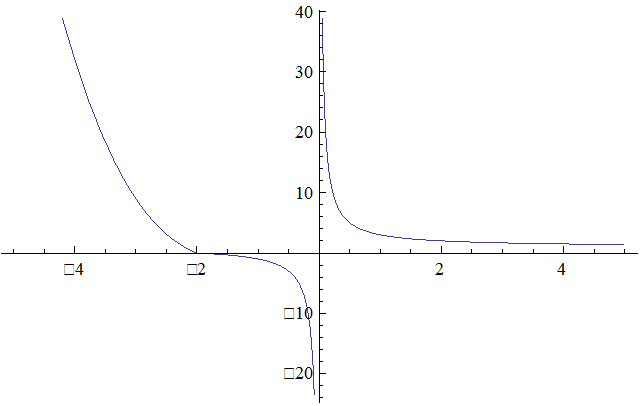The line x=a is a vertical asymptote of the function if at least one of the following statements is true: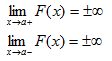Function is defined everywhere except x=0
Let&rsquo;s find limit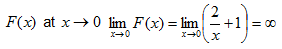Therefore we have the vertical asymptote x=0
Horizontal asymptotes are horizontal lines that the graph of the function approaches as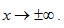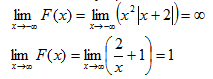Therefore we have the horizontal asymptote y=1
When a linear asymptote is not parallel to the x- or y-axis, it is called an oblique asymptote or slant asymptote.
A function f(x) is asymptotic to the straight line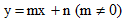if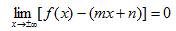Under the function schedule we see that others asymptotes are not present.
In other points it isn&rsquo;t asymptotes because there function is continuous.

We have two asymtotes x=0(vertical) and y=1(horizontal).

Need a fast expert's response?

Submit order

and get a quick answer at the best price

for any assignment or question with DETAILED EXPLANATIONS!